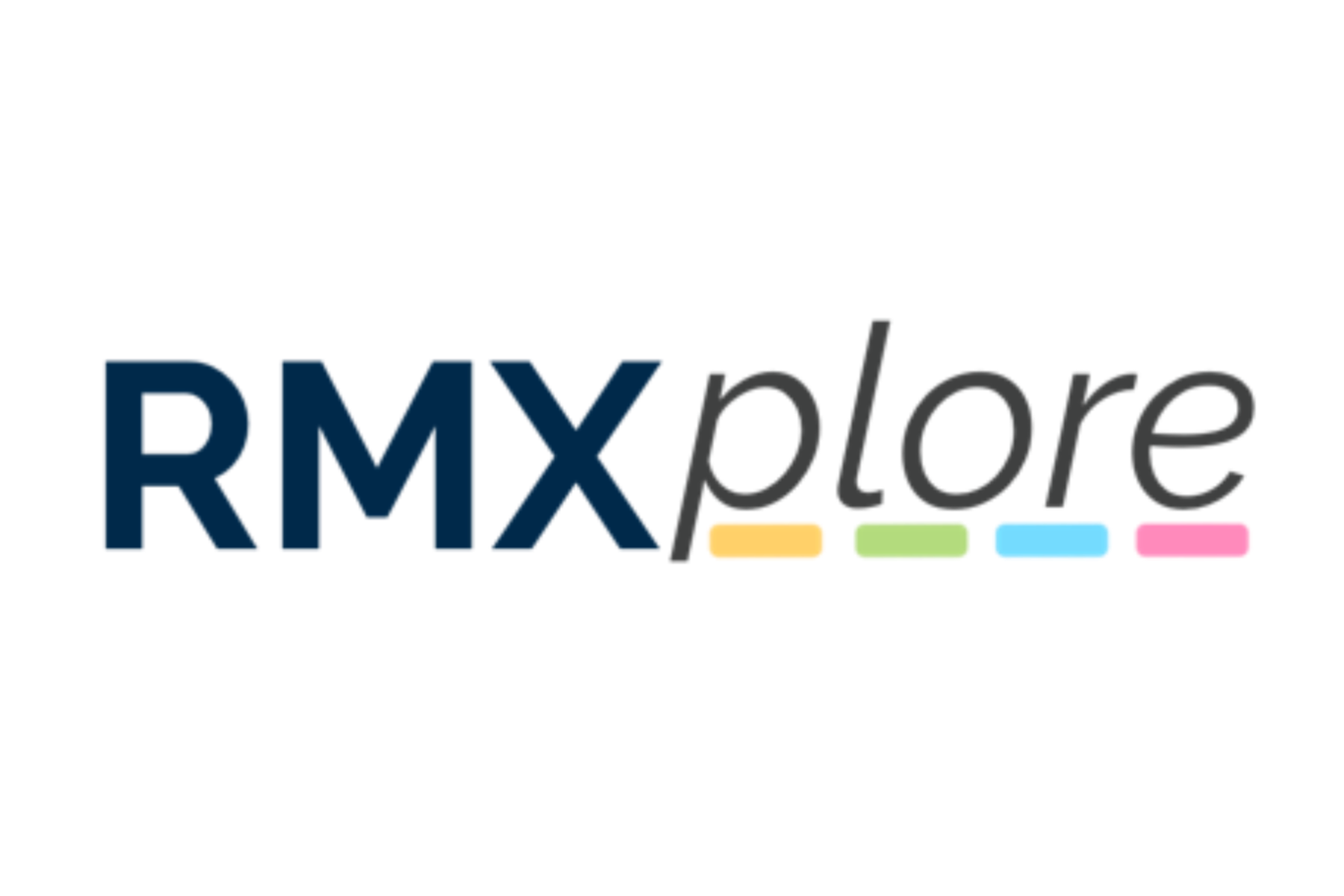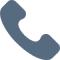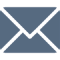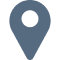# Prealgebra

Please note that RMXplore's Math Bytes Courses are not
currently open for registration
.

### Prealgebra

Coming Soon!
Course Pricing:
• Online : \$260
• Houston Center : \$320
• Cupertino Center : \$400
Course Logistics:
Duration : to be updated
Number of Classes: 5 weeks course
Occurs : to be updated

### Math Byte Course Topics:

Course Topics are organized as six modules with five granular topics covered under each module. Click through below to see all modules/topics for this course.

#### 01 - Angles and Basic Trigonometry• Word problems involving exponents and radicals
• Problem solving using percentage (profit/loss, mixture, etc.)
• Ratio and Proportions (age, population, etc.)
• Rates (Interest, Speed, Work)

#### 02 - Trigonometric Identities & Applications• Word problems involving exponents and radicals
• Problem solving using percentage (profit/loss, mixture, etc.)
• Ratio and Proportions (age, population, etc.)
• Rates (Interest, Speed, Work)

#### 03 - Parametrization and Complex Numbers• Prime numbers
• Divisibility rules
• Prime factorization
• Multiples and Least Common Multiple (LCM)
• Divisors and Greatest Common Divisor (GCD)

#### 04 -Vectors and Matrices• Expressions
• Linear Equations - 1
• Linear Equations - 2
• Inequalities - 1
• Word problems involving linear equations and inequalities

#### 05 - Conics• Angle chasing
• Perimeter and area of shaded regions
• Triangles and Pythagorean Theorem
• Lateral/total surface area and volume of 3D objects

#### 06 - Limits and Continuity• Basic Statistics
• Venn Diagrams
• Counting with Addition and Subtraction
• The Multiplication Principle
• Introduction to Probability